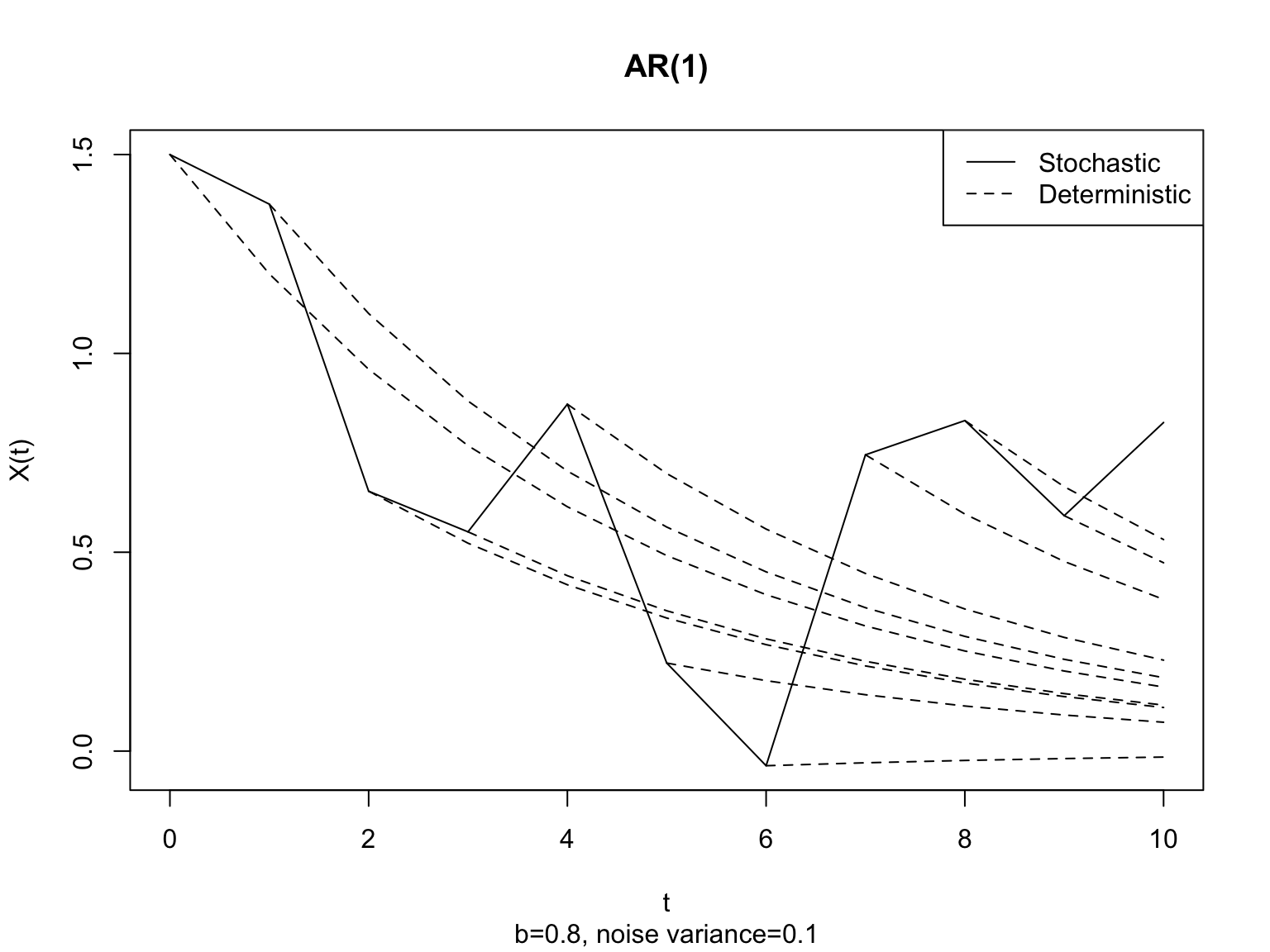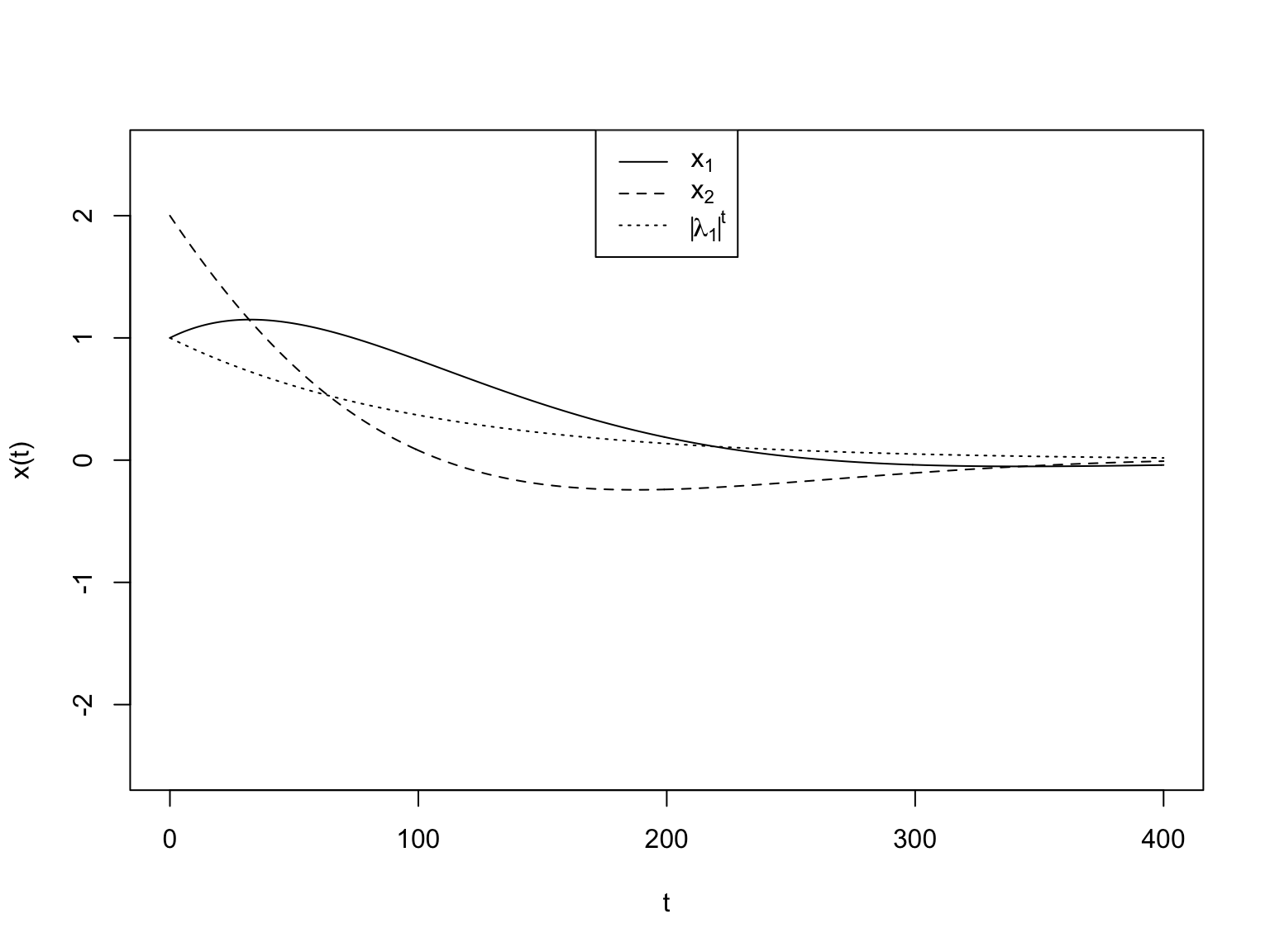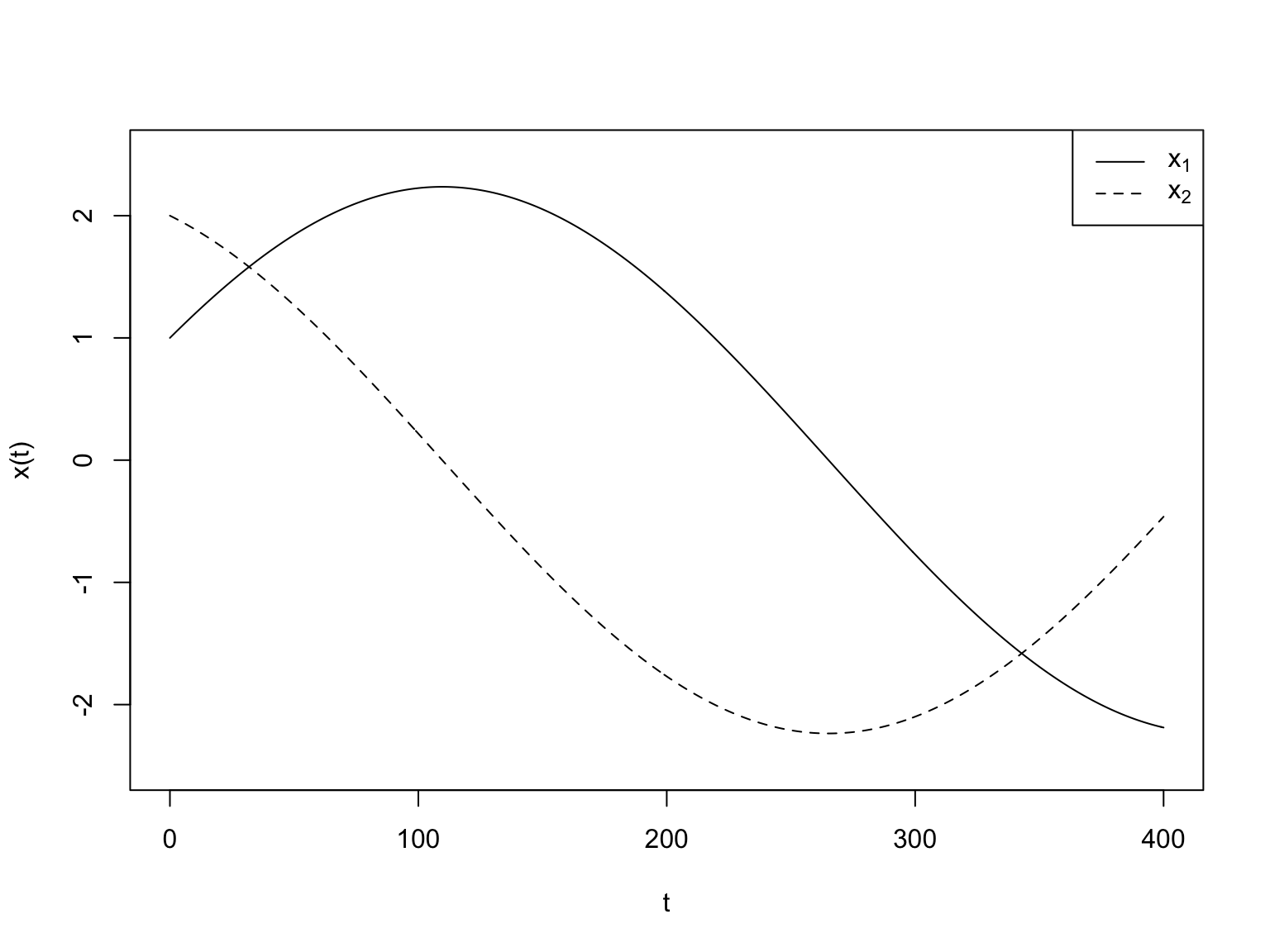# Linear Generative Models for Time Series

16 October 2018

$\newcommand{\Expect}{\mathbb{E}\left[ #1 \right]} \newcommand{\Var}{\mathrm{Var}\left[ #1 \right]} \newcommand{\SampleVar}{\widehat{\mathrm{Var}}\left[ #1 \right]} \newcommand{\Cov}{\mathrm{Cov}\left[ #1 \right]}$

# Previously

• Aimed to be as close to descriptive and exploratory as possible
• Very weak assumptions (like stationarity)
• Or no assumptions (as in PCA)
• Security / robustness / reliability: less can go wrong
• Still able to say something
• Drawbacks:
• Weak on inferences beyond the observables
• Weak on uncertainty
• Going forward:
• Generative models (= distributions over the whole data)
• Uncertainty in inference

# Linear autoregressions

• The first-order linear autoregression, or AR(1):
$\begin{eqnarray} X(t) & = & a + b X(t-1) + \epsilon(t)\\ X(0) & = & \text{some random variable or other} \end{eqnarray}$
• The innovations $$\epsilon(t)$$ are
• All expectation 0
• All uncorrelated with each other
• All uncorrelated with $$X(0)$$
• Typically constant-variance
• To fully specify the model, need to give the distributions of $$X(0)$$ and $$\epsilon$$
• In nice situations, all Gaussian and IID

# Generating a new time series

• Draw $$X(0)$$ from its distribution
• Draw $$\epsilon(1)$$ from its distribution and set $$X(1) \leftarrow a+bX(0)+\epsilon(1)$$
• Iterate:
• Draw $$\epsilon(t)$$ from its distribution
• Set $$X(t) \leftarrow a+bX(t-1)+\epsilon(t)$$

# Unroll the AR(1) a little

$\begin{eqnarray} X(t) & = & a + b X(t-1) + \epsilon(t)\\ & = & a + \epsilon(t) + b(a+b X(t-2) + \epsilon(t-1))\\ & = & a + ba + b^2 a + \ldots + b^{t-1} a + \epsilon(t) + b\epsilon(t-1) + b^2 \epsilon(t-2) + \ldots + b^{t-1} \epsilon(1) + b^t X(0)\\ & = & a\sum_{k=0}^{t-1}{b^k} + \sum_{k=0}^{t}{\epsilon(t-k) b^k} + b^t X(0) \end{eqnarray}$
• At each time, get a random ($$\epsilon(t)$$) plus a deterministic ($$a$$) kick, whose impact is multiplied by $$b$$ at each subsequent time step, forever
• (infinite impulse response)

# Think about the deterministic version

Set $$a=0$$ to simplify book-keeping

$\begin{eqnarray} x(t) & = & b x(t-1)\\ & = & \text{???} \end{eqnarray}$

In-class exercise 1: Write $$x(t)$$ in terms of $$b$$, $$t$$, and $$x(0)$$

# Think about the deterministic version

Set $$a=0$$ to simplify book-keeping

$\begin{eqnarray} x(t) & = & b x(t-1)\\ & = & b^t x(0) \end{eqnarray}$

If $$|b|<1$$ then $$b^t \rightarrow 0$$ as $$t\rightarrow \infty$$

So if $$|b| < 1$$ then $$x(t) \rightarrow 0$$

First-order dynamics are exponential decay to 0 or growth to $$\pm \infty$$

# Adding the noise back in

Constantly being perturbed away from the deterministic path# How would we predict?

Intuitively:

$\hat{X}(t+k) = b^{k} x(t)$

Rigorously:

$\begin{eqnarray} \Expect{X(t+k)|X(t)=x} & = & \Expect{b^k X(t) + \epsilon(t+k) + b \epsilon(t+k-1) + \ldots + b^{k-1}\epsilon(t)|X(t) = x}\\ & = & b^k x + 0 \end{eqnarray}$

because innovations are uncorrelated

$\begin{eqnarray} \Cov{X(t+h), X(t)} & = & \Cov{b^h X(t) + \epsilon(t+h) + b\epsilon(t+h-1) + \ldots + b^{h-1}\epsilon(t), X(t)}\\ & = & b^h \Cov{X(t), X(t)} + 0\\ & = & b^h \Var{X(t)} \end{eqnarray}$

$$\Rightarrow$$ if $$\Var{X(t)}$$ and $$\Expect{X(t)}$$ are constant, this is stationary

# Higher-order autoregressions

$X(t) = a + b_1 X(t-1) + b_2 X(t-2) + \ldots b_p X(t-p) + \epsilon(t)$

• Same idea about how to generate
• Same rules for prediction: $$\Expect{X(t)|X(t-1)=x_1, \ldots X(t-p)=x_p} = a + b_1 x_1 + b_2 x_2 + \ldots b_p x_p$$

Vector autoregression of order 1, or VAR(1)

$\vec{X}(t) = \vec{a} + \mathbf{b} \vec{X}(t-1) + \vec{\epsilon}(t)$

$$\vec{X}(t) =$$ random vector of dimension $$p$$, the state at time $$t$$

$$\vec{a} =$$ deterministic vector of dimension $$p$$

$$\mathbf{b} =$$ deterministic matrix of dimension $$p\times p$$

$$\vec{\epsilon}(t) =$$ random vector of dimension $$p$$, the innovation

Zero out the offset $$\vec{a}$$ for now

$\vec{X}(t) = \mathbf{b} \vec{X}(t-1) + \vec{\epsilon}(t)$

What are the deterministic dynamics?

# Linear dynamical systems in multiple dimensions

$\vec{x}(t) = \mathbf{b}\vec{x}(t-1)$

• Suppose the eigenvectors of $$\mathbf{b}$$ form a basis
• Then for coefficients $$c_1, \ldots c_p$$, $\vec{x}(0) = \sum_{j=1}^{p}{c_j \vec{v}_j}$

# Linear dynamical systems in multiple dimensions

• Dynamics are just multiplying: $\begin{eqnarray} \vec{x}(t) & = & \mathbf{b}\vec{x}(t-1)\\ & = & \mathbf{b}^t \vec{x}(0) \end{eqnarray}$

• In-class exercise: Write $$\vec{x}(t)$$ in terms of the $$c_j$$, the $$\lambda_j$$ and $$\vec{v}_j$$

# Linear dynamical systems in multiple dimensions

• Dynamics are just multiplying: $\begin{eqnarray} \vec{x}(t) & = & \mathbf{b}\vec{x}(t-1)\\ & = & \mathbf{b}^t \vec{x}(0)\\ & = & \sum_{j=1}^{p}{ \lambda^t_j c_j \vec{v}_j} \end{eqnarray}$

# Eigenvalues determine the dynamics of a linear system

The easy case: all eigenvalues $$\lambda_1, \ldots \lambda_p$$ are real

• $$\lambda_j > 1$$: grow along that direction
• $$0 \leq \lambda_j < 1$$: shrink along that direction towards the origin $$\vec{0}$$
• $$\lambda_j < -1$$: flip around the origin, grow in that direction
• $$-1 < \lambda_j \leq 0$$: flip around the origin and shrink

# Eigenvalues determine the dynamics of a linear system

• Some eigenvalues can be complex
• The corresponding coefficients are also complex
• These always come in complex-conjugate pairs
• so the coefficients are complex-conjugate pairs
• The formula $$\vec{x}(t) = \sum_{j=1}^{p}{\lambda^t_j c_j \vec{v}_j}$$ still works
• the imaginary parts always cancel exactly
• Complex eigenvalues $$\Leftrightarrow$$ rotation

# Rotation with complex eigenvalues

b
##       [,1] [,2]
## [1,]  0.99 0.01
## [2,] -0.01 0.99
eigen(b)
## eigen() decomposition
## $values ##  0.99+0.01i 0.99-0.01i ## ##$vectors
##                      [,1]                 [,2]
## [1,] 0.0000000-0.7071068i 0.0000000+0.7071068i
## [2,] 0.7071068+0.0000000i 0.7071068+0.0000000i
Mod(eigen(b)\$values)
##  0.9900505 0.9900505

# Rotation with complex eigenvalues

(x <- matrix(c(1, 2), nrow = 2))
##      [,1]
## [1,]    1
## [2,]    2
b %*% x
##      [,1]
## [1,] 1.01
## [2,] 1.97
b %*% b %*% x
##        [,1]
## [1,] 1.0196
## [2,] 1.9402

# Rotation with complex eigenvalues# Rotation with complex eigenvalues

Reset $$\mathbf{b}^{\prime} = \mathbf{b}/|\lambda_1|$$ so now both eigenvalues have modulus 1# What’s going on here?

• Having both $$x_1(t-1)$$ and $$x_2(t-1)$$ is like having $$x(t-1)$$ and $$x(t-2)$$
• Higher-order ARs are like VARs with extra variables to keep track of past states

# Morals on linear, deterministic dynamical systems

• Find the eigenvalues and eigenvectors
• $$|\lambda_j| < 1 \Rightarrow$$ exponential decay along $$\vec{v}_j$$
• $$|\lambda_j| > 1 \Rightarrow$$ exponential growth
• $$|\lambda_j| = 1 \Rightarrow$$ eternal recurrence
• $$\mathrm{Im}(\lambda_j) \neq 0 \Rightarrow$$ rotations in the space spanned by those eigenvectors
• Higher-order dependence on the past $$\Rightarrow$$ first-order dependence with extra memory variables

• If the eigenvalues are less than 1, $$\mathbf{b}\vec{X}(t-1)$$ has less variance than $$\vec{X}(t-1)$$
• The innovation contributes some extra variance
• To be stationary, the shrinkage has to exactly balance the new variance

# Summarizing

• Autoregressive models are linear dynamical systems plus noise
• Linear dynamics are governed by the eigenvalues and eigenvectors of the linear operator
• The noise perturbs away from the exact linear dynamics
• Stationarity means that the perturbations have to counter-balance the dynamics
• What I haven’t told you: how to estimate these models

# Backup: VAR(1) vs. 2nd-order dynamics

Remember that sine and cosine waves obey $$\frac{d^2 x}{dt^2}(t) = - \omega^2 x(t)$$

Say $$x_1(t)$$ is a sine wave, and define $$x_2(t) = dx_1/dt$$

$\left[ \begin{array}{c} \frac{dx_1}{dt} \\ \frac{dx_2}{dt} \end{array}\right] = \left[ \begin{array}{c} x_2 \\ -x_1 \end{array}\right] = \left[\begin{array}{cc} 0 & 1 \\ -1 & 0\end{array}\right] \left[\begin{array}{c} x_1 \\ x_2 \end{array}\right]$

# Backup: VAR(1) vs. 2nd-order dynamics

Fix a small time increment $$h$$

$\left[ \begin{array}{c} \frac{x_1(t) - x_1(t-h)}{h} \\ \frac{x_2(t)-x_2(t-h)}{h} \end{array}\right] = \left[ \begin{array}{c} x_2(t-h) \\ -x_1(t-h) \end{array}\right]$

# Backup: VAR(1) vs. 2nd-order dynamics

$\left[ \begin{array}{c} x_1(t) - x_1(t-h) \\ x_2(t)-x_2(t-h) \end{array}\right] = \left[ \begin{array}{c} hx_2(t-h) \\ -hx_1(t-h) \end{array}\right]$

# Backup: VAR(1) vs. 2nd-order dynamics

$\left[ \begin{array}{c} x_1(t) \\ x_2(t) \end{array}\right] = \left[ \begin{array}{c} x_1(t-h) + hx_2(t) \\ x_2(t-h) -hx_1(t) \end{array}\right]$

# Backup: VAR(1) vs. 2nd-order dynamics

$\left[ \begin{array}{c} x_1(t) \\ x_2(t) \end{array}\right] = \left[ \begin{array}{cc} 1 & h \\ -h & 1 \end{array}\right] \left[\begin{array}{c} x_1(t-h) \\ x_2(t-h) \end{array}\right]$

# Backup: VAR(1) vs. 2nd-order dynamics

• The extra variable $$x_2$$ helps $$x_1$$ keep track of where it is in its cycle
• Higher-order dynamics = first-order dynamics with extra variables
• Tweaking to have $$|\lambda| = 1$$ gives an exact cycle
• Complex eigenvalues off the unit = cycle $$\times$$ exponential growth or decay

# Backup: the intercept and re-centering

• In 1D: if $$x(t) = a + bx(t-1)$$ and $$y(t)=x(t) - \frac{a}{b-1}$$, then $$y(t) = by(t-1)$$
$\begin{eqnarray} x(t) & = & a+bx(t-1) ~ \text{(assumption)}\\ x(t) - c & = & b(x(t-1) - c) ~\text{(asserted to find right} ~ c)\\ -c & = & -a -bc ~\text{(subtract 2nd line from 1st)}\\ (b-1)c & = & -a ~ \text{(re-arrange)} \end{eqnarray}$
• In multiple dimensions:
$\begin{eqnarray} \vec{x}(t) & = & \vec{a} + \mathbf{b}\vec{x}(t-1)\\ \vec{x}(t) - \vec{c} & = & \mathbf{b}(\vec{x}(t-1)-\vec{c})\\ (\mathbf{I} - \mathbf{b})\vec{c} & = & \vec{a} \end{eqnarray}$
• Always has a solution $$\vec{c}$$ if $$(\mathbf{I}-\mathbf{b})$$ is invertible
• or if $$\vec{a}$$ is in the range space of $$\mathbf{b}$$